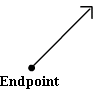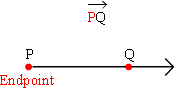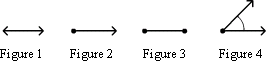Definition Of Ray

A ray is a part of a line that begins at a particular point (called the endpoint) and extends endlessly in one direction. A ray is also called half-line.How do we name a Ray?

A ray is named based on the direction in which it extends.
A ray is named with its endpoint in the first place, followed by the direction in which its moving.
In the example shown below, P is the endpoint and Q is the point towards which the ray extends. So, the ray PQ is represented as:Look at another example. This ray will be called QP as it starts at Q and extends towards P. So, the ray QP is represented as:Solved Example on Ray

Ques: Which of the following is a ray?Solution:

Step 1: The definition of a ray states that "A ray is a part of a line that begins at a particular point (called the endpoint) and extends endlessly in one direction."
Step 2: As per the defition of a ray, among the figures given only Figure 2 represents a ray